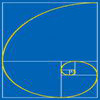Search by Topic

Resources tagged with Golden ratio similar to Mixing More Paints:

Filter by: Content type:
Age range:
Challenge level:

There are 7 results

Broad Topics > Fractions, Decimals, Percentages, Ratio and Proportion > Golden ratioPent

Age 14 to 18 Challenge Level:

The diagram shows a regular pentagon with sides of unit length. Find all the angles in the diagram. Prove that the quadrilateral shown in red is a rhombus.Golden Thoughts

Age 14 to 16 Challenge Level:

Rectangle PQRS has X and Y on the edges. Triangles PQY, YRX and XSP have equal areas. Prove X and Y divide the sides of PQRS in the golden ratio.Pentakite

Age 14 to 18 Challenge Level:

ABCDE is a regular pentagon of side length one unit. BC produced meets ED produced at F. Show that triangle CDF is congruent to triangle EDB. Find the length of BE.Darts and Kites

Age 14 to 16 Challenge Level:

Explore the geometry of these dart and kite shapes!Whirling Fibonacci Squares

Age 11 to 16

Draw whirling squares and see how Fibonacci sequences and golden rectangles are connected.Leonardo of Pisa and the Golden Rectangle

Age 7 to 16

Leonardo who?! Well, Leonardo is better known as Fibonacci and this article will tell you some of fascinating things about his famous sequence.The Golden Ratio, Fibonacci Numbers and Continued Fractions.

Age 14 to 16

An iterative method for finding the value of the Golden Ratio with explanations of how this involves the ratios of Fibonacci numbers and continued fractions.